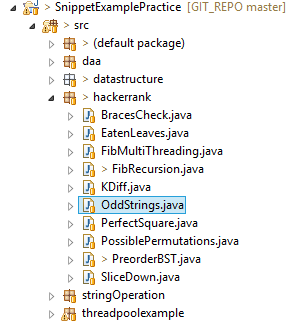# Even or Odd String Interview Question Hacker Rank

Char^Number   X Char^Number    X   (All character of String)  +

[ Char^Number  X  Char^Number   X   (All character of String)  +

So you just need to identify whether there is any even number present in the multiplication.It is asked in the coding challenge taken through Hacker Rank.

=  (97^2  X   98^2   X    99^2)  + (98^2   X   100^2)

] is Even Number or Odd Number ?

So you need to compute the odd count.

Goal: To determine if it is a even or odd string.

Verify preorder sequence of Binary Search Tree (BST) Interview Question Hacker Rank

So you do not need to compute the powers.

Even or Odd String Interview Question Hacker Rank

public class OddStrings public static void main(String args[]) String data[] = aceace,ceceaa,abdbdbdbakjkljhkjh; System.out.println(checkComputation(data)); String data2[] = azbde,abcher,acegk; System.out.println(checkComputation(data2)); public static boolean checkComputation(String data[] ) boolean isStringEvenArray[] = new boolean[data.length] ; int tempVal; boolean isEvenPresent = false; for(int i=0; idata.length;i++) isEvenPresent= false; for(int j=0; jdata[i].length();j++ ) tempVal = (int) data[i].charAt(j); if(tempVal%2==0) isEvenPresent = true; isStringEvenArray[i] = isEvenPresent; int oddCount=0; for(int i=0; idata.length; i++) if(!isStringEvenArray[i]) oddCount++; if(oddCount%2!=0)return false; else return true;

Below is the project structure and source code for the same:

Calculate Fibonacci number in java using Recursion and Multi Threading

1. Even^AnyNumber = Even & Odd^AnyNumber = Odd

3. For sum: if Odd numbers occur odd times, final sum will be Odd.

=  ( 9409 X 9604 X 9801 )  + ( 9604 X 10000)

Here Char[i] is the ASCII value of character.

Input: Number and Array of String containing lower caseThe catch here is not to compute complex mathematical formulas. There are very basic mathematical checks that needs to be followed to compute if this whole sum is even or odd.

When Binary Search Tree can perform worst?